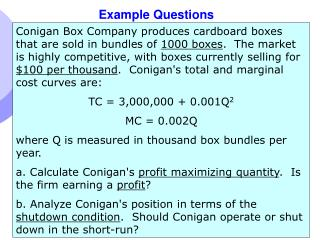DownloadDownload PresentationExample Questions

# Example Questions

Télécharger la présentation## Example Questions

- - - - - - - - - - - - - - - - - - - - - - - - - - - E N D - - - - - - - - - - - - - - - - - - - - - - - - - - -
##### Presentation Transcript

1. Example Questions Conigan Box Company produces cardboard boxes that are sold in bundles of 1000 boxes. The market is highly competitive, with boxes currently selling for \$100 per thousand. Conigan's total and marginal cost curves are: TC = 3,000,000 + 0.001Q2 MC = 0.002Q where Q is measured in thousand box bundles per year. a. Calculate Conigan's profit maximizing quantity. Is the firm earning a profit? b. Analyze Conigan's position in terms of the shutdown condition. Should Conigan operate or shut down in the short‑run? Chapter 8

2. Solution • a. Given the competitive nature of the industry, Conigan should equate P to MC. • 100 = 0.002Q • Q = 50,000 • To determine profit: • = TR – TC TR = PQ = (\$100) (50,000) = 5,000,000 TC = 3,000,000 + 0.001Q2 = 3,000,000 + 0.001(50,000)2 TC = 5,500,000  = 5,000,000 - 5,500,000  = - 500,000 Coningan is losing 500,000 per year Chapter 8

3. b. To determine if the firm should operate on shutdown, we must compare P to AVC AVC = TVC / Q TVC = TC – TFC TVC = 5,500,000 – 3, 000,000 TVC = 2,500,000 AVC = 2,500,000 / 50,000 AVC = \$ 50 So, AVC = \$ 50 and P = \$100, the firm should operate since P > AVC Chapter 8

4. A competitive firm sells its product at a price of \$.10 per unit. Its total and marginal cost functions are: TC = 5 ‑ 0.5Q + 0.001Q2 MC = -0.5 + 0.002Q, where TC is total cost (\$) and Q is output rate (units per time period). 1. Determine the output rate that maximizes profit or minimizes losses in the short‑term. If input prices increase and cause the cost functions to become TC = 5 ‑ 0.10Q + 0.002Q2MC = -0.10 + 0.004Q, 2. What will the new equilibrium output rate be? Explain what happened to the profit maximizing output rate when input prices were increased. Chapter 8

5. TR = PQ • = 0.10 Q • MR = 0.10 • TC = 5 – 0.5 Q + 0.001 Q2 • MC = - 0.5 + 0.002 Q • MC = MR • - 0.5 + 0.002 Q = 0.10 • Q = 75 • b. MC = MR • MC = -0.10 + 0.004Q = 0.10 = MR • Q = 50 • As a result of the increase in input costs, the firm's marginal cost increased. This caused the intersection of MC to occur at the lower production rate, 50 vs. 75. This also reduced the firm's level of profit. Chapter 8

6. The market demand for a carpet has been estimated as: P = 40 ‑ 0.25Q, where P is price (\$/yard) and Q is rate of sales (hundreds of yards per month). The market supply is expressed as: P = 5.0 + 0.05Q. A typical firm in this market has a total cost function given as: C = 100 ‑ 20.0q + 2.0q2. a. Determine the equilibrium market output rate and price. b. Determine the output rate for a typical firm. c. Determine the rate of profit (or loss) earned by the typical firm Chapter 8

7. Equate supply to demand to get Q. • SS = DD • 40 – 0.25 Q = 5.0 + 0.05 Q • 0.30 Q = 35 • Q = 116.7 (hundred of yards per month) • P = 40 – 0.25 (116.7) • = \$ 10.825 / yard • b. The typical firm produces where P = MC • MC = - 20 +4 q • 10.825 = - 20 + 4 q • q = 7.71 ((hundred of yards per month) Chapter 8

8. c. The profit rate is as follows: R (Q) = P Q = (10.825 ) ( 7.71) = 83.461 TC = 100 – 20 (7.71) + 2 (7.71) 2 = 64.69  = \$18.77 ( hundreds per month) Chapter 8